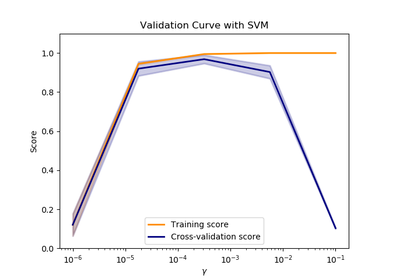# `sklearn.model_selection`.validation_curve¶

`sklearn.model_selection.``validation_curve`(estimator, X, y, param_name, param_range, groups=None, cv=None, scoring=None, n_jobs=1, pre_dispatch=’all’, verbose=0)[source]

Validation curve.

Determine training and test scores for varying parameter values.

Compute scores for an estimator with different values of a specified parameter. This is similar to grid search with one parameter. However, this will also compute training scores and is merely a utility for plotting the results.

Read more in the User Guide.

Parameters: estimator : object type that implements the “fit” and “predict” methods An object of that type which is cloned for each validation. X : array-like, shape (n_samples, n_features) Training vector, where n_samples is the number of samples and n_features is the number of features. y : array-like, shape (n_samples) or (n_samples, n_features), optional Target relative to X for classification or regression; None for unsupervised learning. param_name : string Name of the parameter that will be varied. param_range : array-like, shape (n_values,) The values of the parameter that will be evaluated. groups : array-like, with shape (n_samples,), optional Group labels for the samples used while splitting the dataset into train/test set. cv : int, cross-validation generator or an iterable, optional Determines the cross-validation splitting strategy. Possible inputs for cv are: None, to use the default 3-fold cross validation, integer, to specify the number of folds in a (Stratified)KFold, An object to be used as a cross-validation generator. An iterable yielding train, test splits. For integer/None inputs, if the estimator is a classifier and `y` is either binary or multiclass, `StratifiedKFold` is used. In all other cases, `KFold` is used. Refer User Guide for the various cross-validation strategies that can be used here. scoring : string, callable or None, optional, default: None A string (see model evaluation documentation) or a scorer callable object / function with signature `scorer(estimator, X, y)`. n_jobs : integer, optional Number of jobs to run in parallel (default 1). pre_dispatch : integer or string, optional Number of predispatched jobs for parallel execution (default is all). The option can reduce the allocated memory. The string can be an expression like ‘2*n_jobs’. verbose : integer, optional Controls the verbosity: the higher, the more messages. train_scores : array, shape (n_ticks, n_cv_folds) Scores on training sets. test_scores : array, shape (n_ticks, n_cv_folds) Scores on test set.

Notes

## Examples using `sklearn.model_selection.validation_curve`¶Plotting Validation Curves